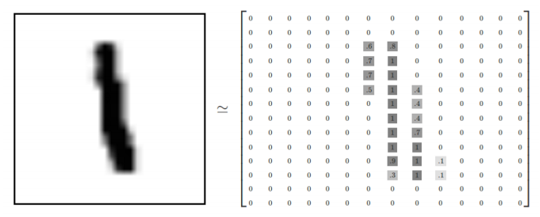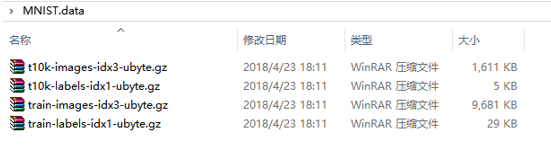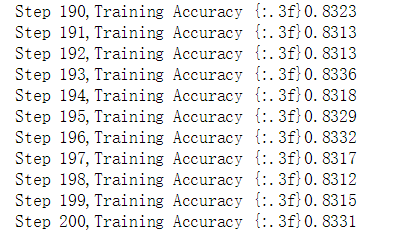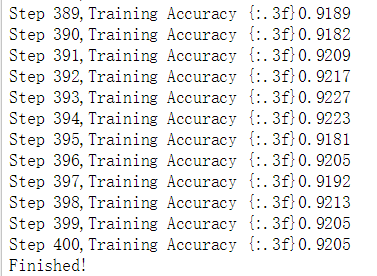# Tensorflow实现MNIST手写数字识别

### 一 、MNIST手写数字数据集介绍

MNIST手写数字数据集来源于是美国国家标准与技术研究所，是著名的公开数据集之一，通常这个数据集都会被作为深度学习的入门案例。数据集中的数字图片是由250个不同职业的人纯手写绘制，数据集获取的网址为：http://yann.lecun.com/exdb/mnist/。（下载后需解压）### 二 、网络结构的设计

 mnist_data=input_data.read_data_sets("MNIST.data",one_hot=True) batch_size=100 batch_num=mnist_data.train.num_examples//batch_sizex = tf.placeholder(tf.float32,[None,784]) y = tf.placeholder(tf.float32,[None,10]) 

 weights = { 'hidden_1': tf.Variable(tf.random_normal([784, 256])), 'out': tf.Variable(tf.random_normal([256, 10])) } biases = { 'b1': tf.Variable(tf.random_normal()), 'out': tf.Variable(tf.random_normal()) } 

 def neural_network(x): hidden_layer_1 = tf.add(tf.matmul(x, weights['hidden_1']), biases['b1']) out_layer = tf.matmul(hidden_layer_1, weights['out']) + biases['out'] return out_layer 

 #调用神经网络 result = neural_network(x) #预测类别 prediction = tf.nn.softmax(result) #平方差损失函数 loss = tf.reduce_mean(tf.square(y-prediction)) #梯度下降法 train_step = tf.train.GradientDescentOptimizer(0.2).minimize(loss) #预测类标 correct_pred = tf.equal(tf.argmax(y,1),tf.argmax(prediction,1)) #计算准确率 accuracy = tf.reduce_mean(tf.cast(correct_pred,tf.float32)) #初始化变量 init = tf.global_variables_initializer() 

 step_num=400 with tf.Session() as sess: sess.run(init) for step in range(step_num+1): for batch in range(batch_num): batch_x,batch_y = mnist_data.train.next_batch(batch_size) sess.run(train_step,feed_dict={x:batch_x,y:batch_y}) acc = sess.run(accuracy,feed_dict={x:mnist_data.test.images,y:mnist_data.test.labels}) print("Step " + str(step) + ",Training Accuracy "+ "{:.3f}" + str(acc)) print("Finished!")#预测类别 prediction = tf.nn.softmax(result) #交叉熵损失函数 loss = tf.reduce_mean(tf.nn.softmax_cross_entropy_with_logits(labels=y,logits=prediction)) #梯度下降法 train_step = tf.train.GradientDescentOptimizer(0.2).minimize(loss) #预测类标 correct_pred = tf.equal(tf.argmax(y,1),tf.argmax(prediction,1)) #计算准确率 accuracy = tf.reduce_mean(tf.cast(correct_pred,tf.float32))#Adam优化算法 train_step = tf.train.AdamOptimizer(1e-2).minimize(loss) 

Dropout是在每次神经网络的训练过程中，使得部分神经元工作而另外一部分神经元不工作。而测试的时候激活所有神经元，用所有的神经元进行测试。这样便可以有效的缓解过拟合，提高模型的准确率。具体代码如下所示：

 def neural_network(x): hidden_layer_1 = tf.add(tf.matmul(x, weights['hidden_1']), biases['b1']) L1 = tf.nn.tanh(hidden_layer_1) dropout1 = tf.nn.dropout(L1,0.5) out_layer = tf.matmul(dropout1, weights['out']) + biases['out'] return out_layer